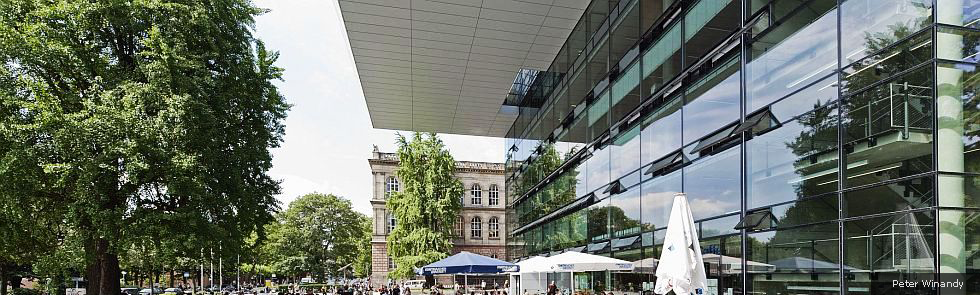Kategorien
Seiten
-## Metric-Based Anisotropic Adaptation for Optimal Petrov-Galerkin Methods

09. Oktober 2018 | von

Prof. Georg May, Ph.D.Certain Petrov-Galerkin methods (optimal PG schemes) deliver inherently stable formulations of variational problems on a given mesh, by selecting appropriate pairs of trial and test spaces. Depending on the choice of norms, quasi-optimal and robust schemes, e.g. for singularly perturbed problems, with stability constants independent of the perturbation parameter, may be obtained.

Optimal PG schemes can be interpreted as minimal residual methods, minimizing the residual in the dual norm (of the test space). In fact, some schemes compute the Riesz representation of the dual residual as part of the solution methodology. In this sense, these optimal Petrov-Galerkin schemes come with a built-in error estimate. Adaptation has therefore been traditionally strongly linked with optimal PG schemes.

Conventionally, adaptation means locally refining the given discrete mesh. A step forward would be the global optimization of the mesh with respect to the inherent error information coming from the optimal Petrov-Galerkin framework. In this context, metric-based continuous mesh models are ideal candidates. A metric-conforming mesh is a triangulation whose elements are (nearly) equilateral under the Riemannian metric induced the continuous mesh model. (Analytic) optimization techniques, based on calculus of variations, may be applied to the metric field, rather than the discrete mesh. The task to produce a mesh is thus delayed until an optimized metric is available.

The error models driving previous continuous-mesh optimization methods need to be adapted to the error representation coming directly from the optimal PG methodology. The goal is to formulate the correct continuous-mesh error models for the optimal Petrov-Galerkin methodology. Nontrivial applications, such as highly convection-dominated problems will be considered to validate the concept.

Kommentare sind geschlossen.